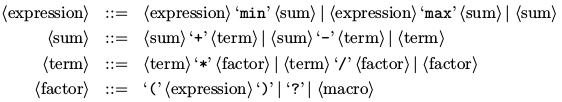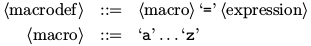### J - Java2016

##### Languages: C, C++, Java, Pascal, ... (details)

John likes to learn esoteric programming languages. Recently he discovered the probabilistic programming language Java2K. Built-in functions of Java2K have only a certain probability to do whatever you intend them to do.

The Java2K programming is very hard, so John designed a much simpler language for training: Java2016. Built-in operators of Java2016 are deterministic, while their operands are random. Each value in Java2016 is a positive integer in the range $0..255$, inclusive.

Java2016 supports six operators of three precedencies:Minimum ('$\texttt{min}$') and maximum ('$\texttt{max}$') operators are defined as usual. Addition ('$\texttt{+}$'), subtraction ('$\texttt{-}$') and multiplication ('$\texttt{*}$') are defined modulo 256. The result of the division ('$\texttt{/}$') is rounded towards zero. If the divider is zero, the program crashes. The argument of the operator is a result of another operator, evenly distributed random value ('$\texttt{?}$'), or macro substitution.

For instance, the probability that "$\texttt{?/?/?}$" is evaluated to zero is $98.2\%$, while the probability of the crash is $0.8\%$.

The Java2016 program consists of zero or more macro definitions, followed by the resulting expression. Each macro definition has a form ofThe macro should be defined before the first use. It may not be redefined. The macro is expanded to its definition on each use. For instance,
$\\\verb| a = ? max ?|$
$\\\verb| (a max a) / a|$
is expanded to "$\\\verb|((? max ?) max (? max ?)) / (? max ?)|$".

John is going to add probabilistic constants to Java2016, so for each possible constant value he needs a program that successfully evaluates to this value with at least one-half probability. Crashes are counted toward failures.

#### Input

The input contains a single integer $c$ — the target constant ($0 \le c \le 255$).

#### Output

Output a Java2016 program that successfully evaluates to constant $c$ with probability no less than $1/2$. The total length of the program should not exceed $256$ characters (excluding spaces).

#### Sample test(s)

Input
0
Output
? /?/ ?
Input
1
Output
a = ? max ? (a max a) / a